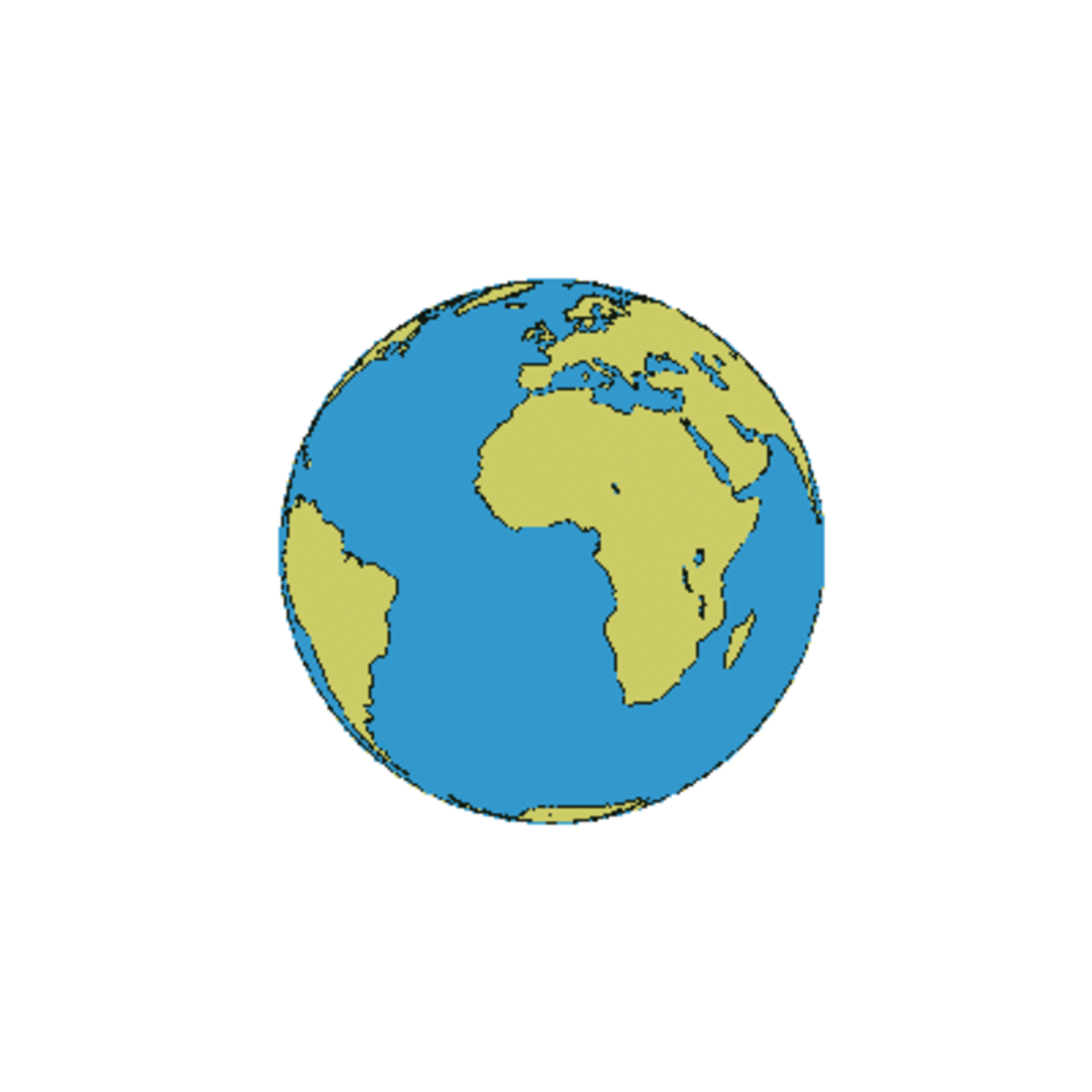Agency
1738 views 5 likes

A satellite can remain in the same orbit for a long period of time as the gravitational pull of the Earth provides a balance to the centrifugal force. As the satellites are in orbit outside the atmosphere there is no air resistance, and therefore, according to the law of inertia, the speed of the satellite is constant resulting in a stable orbit around the Earth for many years.

Gravitational pull diminishes as one travels further from the Earth, whereas the centrifugal force increases as the orbital velocity is increased. Therefore, a satellite in low orbit, typically about 800km from the Earth is exposed to an immense gravitational pull and has to move at considerable speed to generate a corresponding centrifugal force. Therefore, there is a direct connection between the distance to the Earth and the orbital velocity of the satellite. At a distance of 36000km, the orbiting time is 24 hours corresponding to the Earth's rotation time. At this distance a satellite above the Equator will be stationary in relation to the Earth.